## 16.1 Some Rules for Approximate Evaluation: the Trapezoid Rule

### 16.1.1 Trapezoid Rule

We can approximate an integral by choosing n, iding the integration interval into n parts and using a Riemann sum as our approximation.

Let Ln be the result of computing the left Riemann sum in this way, and Rn be the right Riemann sum. The "trapezoid rule" is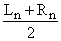.

Here are explicit formulae for these approximate sums: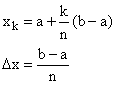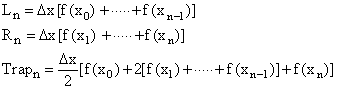### 16.1.2 Simpson's Rule

Simpson's rule works only for n even and gives different weight to the f(xk) depending on the evenness or oddness of k: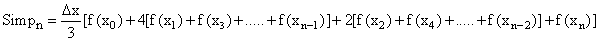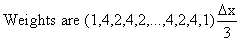Why use Simpson's rule